# [4.NF.3c-1.0] Mixed Numbers with Like Denominators - Common Core Standard

##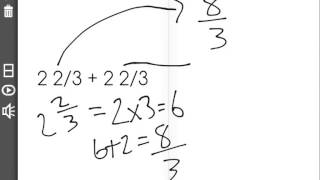By Freckle education

Add and subtract mixed numbers with like denominators, e.g., by replacing each mixed number with an equivalent fraction, and/or by using properties of operations and the relationship between addition and subtraction.# Multiplying fractions by whole numbers 4.NF.B.4 4.NF.4

##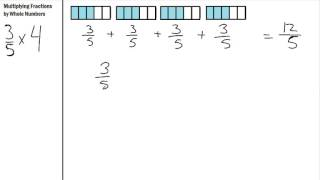By S Hart

Multiplying Fractions by Whole numbers using repeated addition# identfying even and odd numbers using visuals 2.OA.3

##By Tenmarks Amazon

numbers using objects grouped into groups, arrays, paired objects, and number lines. Discusses that even numbers can be split into 2 groups.# Multiplying Fractions by Whole Numbers

##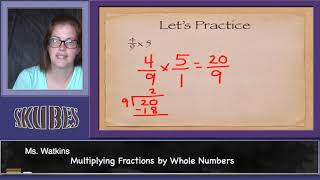By Skubes ed

Understand a multiple of a/b as a multiple of 1/b, and use this understanding to multiply a fraction by a whole number.# Dividing Mixed Numbers

##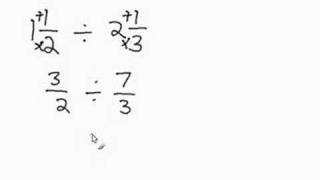By Davitily

The instructor uses an electronic chalkboard to demonstrate how to divide mixed numbers. One example is modeled using a step by step approach.# Multiplying and dividing negative numbers

##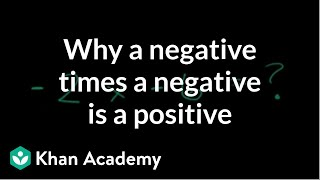By Khan Academy

Use the distributive property to understand the products of negative numbers.# Understanding Numbers and Place Value (4.NBT.2)

##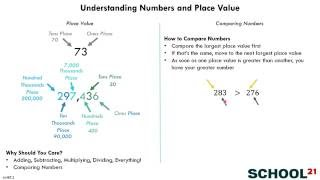By School 21

Read and write multi-digit whole numbers using base-ten numerals, number names, and expanded form# Multiplying Fractions by Whole Numbers

##By Skubes ed

Understand a multiple of a/b as a multiple of 1/b, and use this understanding to multiply a fraction by a whole number# Multiplying and dividing negative numbers

##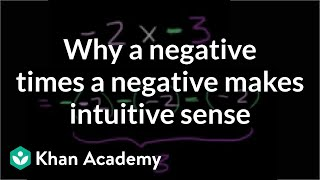By Khan Academy

Use the repeated addition model of multiplication to give an understanding of multiplying negative numbers.# Grade 2 Math 9.5, Understanding place value (three-digits)

##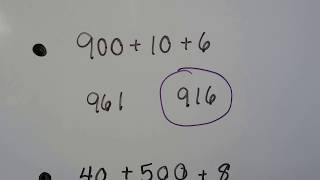By Joann's School

How each digit in a three-digit number has a different value as hundreds, tens, or ones. Expanded form of a three-digit number. Identifying the value of a digit in a three-digit number. Counting by hundreds, then writing the missing number by using a pattern. Writing a number represented by models of hundreds, tens, and ones# Multiplying 4 digits by 1 digit with visual models

##By Khan Academy

It's helpful sometimes to use a grid when multiplying multi-digit numbers. Here's an example using a 4 digit number times a 1 digit number.# Estimate the Product of Mixed Numbers

##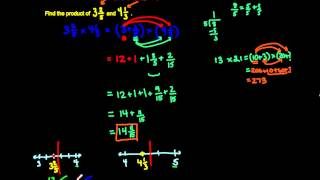By MathwithMrAlmeida

Use your number sense to estimate what the product will be. A great skill to have to check your work!# Multiplying Fractions by Whole Numbers in Word Problems

##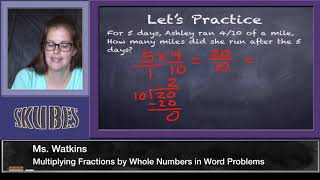By Skubes ed

Solve real world problems involving multiplication of fractions and mixed numbers, e.g., by using visual fraction models or equations to represent the problem# Ex 2: Set Problem Solving - Find the Number of Element in the Union of Two Sets Using a Formula

##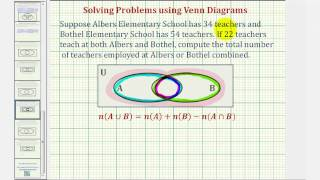By Mathispower4u

Ex 2: Set Problem Solving - Find the Number of Element in the Union of Two Sets Using a Formula# [5.NBT.2-1.0] Multiplying/Dividing by 10 - Common Core Standard - Practice Problem

##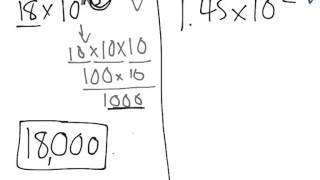By Front Row

Explain patterns in the number of zeros of the product when multiplying a number by powers of 10, use whole-number exponents to denote powers of 10# Adding Mixed Numbers with Uncommon Denominators

##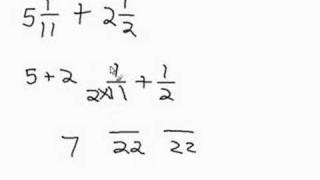By Davitily

The instructor uses an electronic chalkboard to demonstrate how to add mixed numbers with uncommon denominators. One example is modeled using astep by step approach to walk the learner through this process of adding the whole numbers first and then finding the common denominator to add the remaining fractions together.# Multiplying by 10

##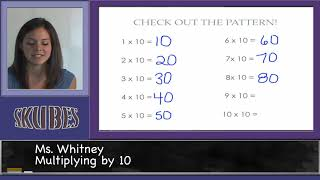By Skubes ed

Multiply one-digit whole numbers by multiples of 10 in the range 10–90 (e.g., 9 × 80, 5 × 60) using strategies based on place value and properties of operations.# [4.NF.4b-1.0] Multiplying Fractions - Common Core Standard

##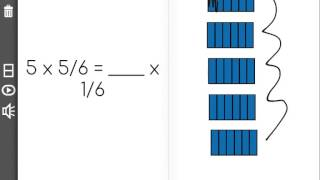By Freckle education

Understand a multiple of a/b as a multiple of 1/b, and use this understanding to multiply a fraction by a whole number# Steps to Multiply Big Numbers Using the Standard Method

##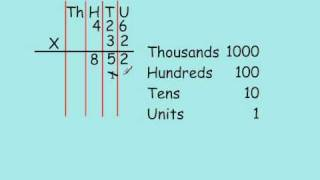By Joseph Gliddon

This video goes step by step though a standard method to multiply a 3 digit number by a 2 digit number. Examples are shown of step by step process for solving multiplication of multi-digit numbers.# [4.NF.4c-1.0] Word Problems: Multiplying - Common Core Standard

##By Freckle education

Solve word problems involving multiplication of a fraction by a whole number, e.g., by using visual fractions models and equations to represent the problem.